# Crack the top 40 C++ coding interview questions

Oct 29, 2020 - 20 min readRyan Thelin## C++ from Scratch Series

• ##### Top 40 C++ Coding Interview Questions

C++ is a popular OOP programming language used across the tech industry. Companies like Microsoft, LinkedIn, Amazon, and PayPal list C++ as their main programming language with others for coding interviews.

Overall, C++ is a must-have skill for modern developers interested in these large companies.

Today, we’ll go through the top 40 C++ coding interview questions used to test C++. By the end, you’ll have the confidence and hands-on experience to approach any C++ interview confidently.

Here’s what we’ll cover today:

## Big O notation questions

### 1. Nested Loop with Addition

Compute the complexity of the following code snippet:

int main(){
int n = 10;
int sum = 0;
float pie = 3.14;

for (int i=1; i<n; i+=3){
cout << pie << endl;
for (int j=1; j<n; j+=2){
sum += 1;
cout << sum << endl;
}
}
}


Solution Explination

On line 6 in the outer loop, int i=1; runs once, i<n; gets executed ${n}/{3}+1$ times and i+=3 is executed ${n}/{3}$ times.

In the inner loop, int j=1; gets executed ${n}/{3}$ times in total. j<n; executes $({n}/{3})$ $*$ $({n}/{2}+1)$ times and j+=2 gets executed $({n}/{3})$ $*$ $({n}/{2})$ times.

Statement Number of Executions
int n = 10; 1
int sum = 0; 1
float pie = 3.14; 1
int i=1; 1
i<n; $n/3$ + 1
i+=3 $n/3$
cout << pie << endl; $n/3$
int j=1; $n/3$
j<n; $({n}/{3})$ $*$ $({n}/{2}+1)$
j+=2 $({n}/{3})$ $*$ $({n}/{2})$
sum += 1; $({n}/{3})$ $*$ $({n}/{2})$
cout << sum << endl; $({n}/{3})$ $*$ $({n}/{2})$

Added together, the runtime complexity is $15+$ $({5n}/3)$ $+(2n^2)/3$

Now convert this to Big O.

1. Drop the leading constants: $n+n^2$
2. Drop lower order terms: $n^2$

### 2. Nested Loop with Multiplication

Find the Big O complexity of the following code:

int main() {
int n = 10; //n can be anything
int sum = 0;
float pie = 3.14;
int var = 1;

while (var < n){
cout << pie << endl;
for (int j=0; j<var; j++)
sum+=1;
var*=2;
}
cout<<sum;
}


Solution Explination

Statement Number of executions
int n = 10; 1
int sum = 0; 1
float pie = 3.14; 1
int var = 1; 1
while(var < n) $log$2$(n)+1$
cout << pie << endl $log$2$(n)$
int j=0; $log$2$(n)$
j<var; $2n$
j++ $2n-1$
sum+=1; $2n-1$
var*=2; $log$2$(n)$
cout<<sum; 1

Runtime complexity: $4+$ $4log$2$(n)$ $+6n$

Big O: $O(n)$

### 3. Common mistakes with nested loop

Find the Big O complexity of the following code:

for( int i=0; i<array.length; i++){
for(int j=0; j<10000; j++)
{
// some useful work done here.
}
}


Solution Explanation

This is a “gotcha” question that tests if you really understand Big O.

Even if they’re nested, the inner loop’s number of executions is not dependent on the input. It will always execute the same number of times.

A common mistake is to answer $O(10000n)$. Big O calculates for asymptotic behavior therefore we remove constants, like 10,000.

The correct answer is then $O(n)$.

### 4. Do-while loop

Find the complexity of the following code:

void averager(int[] A) {

float avg = 0.0f;
int j, count;

for (j = 0; j < A.length; j++) {
avg += A[j];
}

avg = avg / A.length;

count = j = 0;

do {

while (j < A.length && A[j] != avg) {
j++;
}

if (j < A.length) {
A[j++] = 0;
count++;
}
} while (j < A.length);
}

Solution Explanation

The for loop from lines 6-8 calculates the average of the contents of the array. It’s complexity is $O(n)$.

The do-while loop from lines 14-24 is tricky. It may seem that the complexity is $O(n^2)$ because of the nested loops.

In fact ,we have to account for two possibilities.

1. The average calculated for the given input array also occurs in the array.
2. The average is a float and doesn’t appear in the array.

If the average is a float then the nested while loop on lines 16-17 will increment the value of the variable j to the size of the input array. Also, the do-while condition will become false.

The complexity in this case will be $O(n)$

If the average does appear in the array and the array consists of all the same numbers, then the nested while loop of lines 16-17 will not run. The if clause of lines 20-23 will kick-in and increment j to the size of the array as the outer do-while loop iterates.

Hence the overall complexity of the snippet is $O(n)$.

### 5. Recursive Complexity

Find the Big O complexity of the following code:

int recursiveFun1(int n)
{
if (n <= 0)
return 1;
else
return 1 + recursiveFun1(n-1);
}

int recursiveFun2(int n)
{
if (n <= 0)
return 1;
else
return 1 + recursiveFun2(n-5);
}

int recursiveFun3(int n)
{
if (n <= 0)
return 1;
else
return 1 + recursiveFun3(n/5);
}

void recursiveFun4(int n, int m, int o)
{
if (n <= 0)
{
printf("%d, %d\n",m, o);
}
else
{
recursiveFun4(n-1, m+1, o);
recursiveFun4(n-1, m, o+1);
}
}

int recursiveFun5(int n)
{
for (i = 0; i < n; i += 2) {
// do something
}

if (n <= 0)
return 1;
else
return 1 + recursiveFun5(n-5);
}


Solution

For this one, it’s best to break down the problem function by function.

int recursiveFun1(int n)
{
if (n <= 0)
return 1;
else
return 1 + recursiveFun1(n-1);
}


This function is called n times before reaching base case. It therefore has a Big O complexity of $O(n)$.

int recursiveFun2(int n)
{
if (n <= 0)
return 1;
else
return 1 + recursiveFun2(n-5);
}


This function is called n-5 times and simplifies to $O(n)$ with Big O.

int recursiveFun3(int n)
{
if (n <= 0)
return 1;
else
return 1 + recursiveFun3(n/5);
}


The complexity here is $log(n)$ because of every time we divide by 5 before calling the function.

void recursiveFun4(int n, int m, int o)
{
if (n <= 0)
{
printf("%d, %d\n",m, o);
}
else
{
recursiveFun4(n-1, m+1, o);
recursiveFun4(n-1, m, o+1);
}
}


Here the complexity is $O(2^n)$ because each function calls itself twice unless recurred n times.

int recursiveFun5(int n)
{
for (i = 0; i < n; i += 2) {
// do something
}

if (n <= 0)
return 1;
else
return 1 + recursiveFun5(n-5);
}


Finally, the for loop executes $n/2$ times because i iterates by 2 and the recursion takes $n-5$ since the loop is called recursively.

Together, they have a complexity of $2n^2-10n$ or $O(n^2)$.

## Data Structures

### 6. Find the Smallest common number

Problem statement:

“Given three integer arrays sorted in ascending order, return the smallest number that is common in all three arrays. Return -1 if there is no common number.”

Hints:

• The arrays are already sorted so their smallest element is at index 0.
• Use three pointers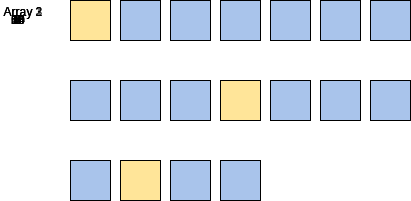Solution and Breakdown

int find_least_common_number(vector<int>& arr1, vector<int>& arr2, vector<int>& arr3) {
int i = 0, j = 0, k = 0;

while(i < arr1.size() && j < arr2.size() && k < arr3.size()) {

// Finding the smallest common number
if((arr1[i] == arr2[j]) && (arr2[j] == arr3[k]))
return arr1[i];

// Let's increment the iterator
// for the smallest value.

if(arr1[i] <= arr2[j] &&  arr1[i] <= arr3[k]) {
i++;
}

else if(arr2[j] <= arr1[i] && arr2[j] <= arr3[k]) {
j++;
}

else if(arr3[k] <= arr1[i] && arr3[k] <= arr2[j]) {
k++;
}
}
return -1;
}

int main(int argc, char* argv[]) {
vector<int> v1 = {6, 7, 10, 25, 30, 63, 64};
vector<int> v2 = {1, 4, 5, 6, 7, 8, 50};
vector<int> v3 = {1, 6, 10, 14};
int result = find_least_common_number(v1, v2, v3);
cout << "Least Common Number: " <<result;
}

Time complexity: $O(n)$

We use three iterators simultaneously to traverse each of the arrays. Each pointer starts at the 0th index because that is the smallest in the list.

The program first looks to see if the 0th element is shared. Then, we’ll return thatvalue.

Otherwise, we’ll see which iterator amongst the three points to the smallest value and will increment that iterator so that it will point to the next index. We have found the solution when all iterators match.

If any of the three iterators reaches the end of the array before its finds a common number, we’ll return -1.

### 7. Move All Zeros to the Beginning of the Array

Problem Statement

“Given an integer array, move all elements that are 0 to the left while maintaining the order of other elements in the array. The array must be modified in-place.”

Hints:

• You cannot use a simple chronological sort as elements other than zeros must maintain their position.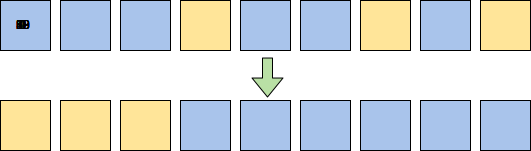Solution and Breakdown

void move_zeros_to_left(int A[], int n) {

if (n < 1) return;

int write_index = n - 1;
int read_index = n - 1;

write_index--;
}

}

while(write_index >= 0) {
A[write_index] = 0;
write_index--;
}
}
int main() {
int v[] = {1, 10, 20, 0, 59, 63, 0, 88, 0};
int n = sizeof(v) / sizeof(v);

cout << "Original Array" <<endl;

for(int x=0 ; x<n; x++) {
cout << v[x];
cout << ", ";
}

move_zeros_to_left(v, n);

cout << endl<< "After Moving Zeroes to Left"<< endl;
for(int i=0 ; i<n; i++) {
cout << v[i];
cout << ", ";
}
}  

Time complexity: $O(n)$

We keep two markers: read_index and write_index. Both start pointed to the end of the array.

While moving read_index towards the start of the array:

If read_index points to 0, we that skip element.

If read_index points to a non-zero value, we write the value at read_index to the write_index and then decrement write_index.

Once read_index reaches the end of the array, we assign zeros based on the location of write_index. Any values on or before write_index must be zeros.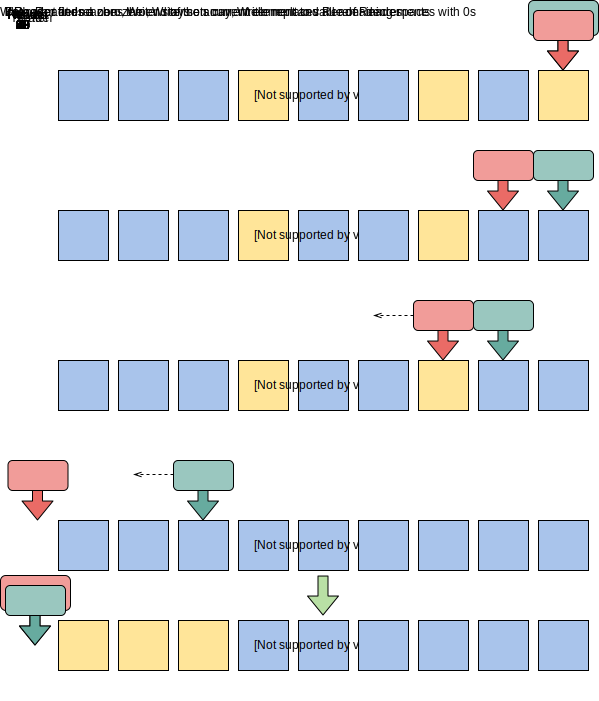### 8. Reverse Even Nodes in a Linked List

Problem Statement

“Given a singly linked list, reverse the nodes at even indices (starting at 1).”

Hint:

• Create a list for even numbers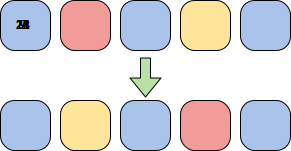Solution and breakdown

// Helper function to merge two lists.
if (list1 == nullptr) {
return list2;
}

if (list2 == nullptr) {
return list1;
}

while (list1->next != nullptr && list2 != nullptr) {
list2 = list2->next;

temp->next = list1->next;
list1->next = temp;
list1 = temp->next;
}

if (list1->next == nullptr) {
list1->next = list2;
}

}

// Let's extract even nodes from the existing
// list and create a new list consisting of
// even nodes. We will push the even nodes at
// head since we want them to be in reverse order.

while (curr != nullptr && curr->next != nullptr) {
curr->next = even->next;

// Push at the head of new list.
even->next = list_even;
list_even = even;

curr = curr->next;
}

// Now, merge the two lists
// Original: 1,2,3,4,5
// Modified original: 1,3,5
// List_even: 4,2
// Merged: 1,4,3,2,5

}

int main() {
vector<int> v1 = {7, 14, 21, 28, 9};

cout << "Original list: ";

cout <<"After reversing even nodes: ";
}

Time Complexity: $O(n)$

We will create two lists comprising of nodes at even and odd indices.

We avoid copying data of elements or reallocating memory to improve efficiency.

We push extracted nodes to the head of list_even to reverse their order while merging.

Once the two lists are in the correct order, we merge their nodes alternately to creat our solution.

### 9. Sort a Linked List Using Merge Sort

Problem Statement

“Given the head pointer of a linked sort, sort the linked list in ascending order using merge sort, and return the new head pointer of sorted linked list.”

Hint:

• Divide and Conquer

Solution and Breakdown

typedef LinkedListNode* node_ptr;

// this method splits linked list in two halves by iterating over whole list
// It returns head pointers of first and 2nd halves of linked lists in first_second
void split_in_half(node_ptr head, pair<node_ptr, node_ptr>& first_second) {
return;
}

// Only one element in the list.
first_second.second = nullptr;
} else {
// Let's use the classic technique of moving two pointers:
// 'fast' and 'slow'. 'fast' will move two steps in each
// iteration where 'slow' will be pointing to middle element
// at the end of loop.
node_ptr slow, fast;

while (fast != nullptr) {
fast = fast->next;

if (fast != nullptr) {
fast = fast->next;
slow = slow->next;
}
}

first_second.second = slow->next;

slow->next = nullptr;
}
}

node_ptr merge_sorted_lists(node_ptr first, node_ptr second) {
if (first == nullptr) {
return second;
}
else if (second == nullptr) {
return first;
}

if (first->data <= second->data) {
first = first->next;
}
else {
second = second->next;
}

while (first != nullptr && second != nullptr) {
node_ptr temp = nullptr;
if (first->data <= second->data) {
temp = first;
first = first->next;
} else {
temp = second;
second = second->next;
}

new_current->next = temp;
new_current = temp;
}

if (first != nullptr) {
new_current->next = first;
} else if (second != nullptr) {
new_current->next = second;
}

}

// No need to sort a single element.
}

// Let's split the list in half, sort the sublists
// and then merge the sorted lists.
pair<node_ptr,node_ptr> first_second;

first_second.first = merge_sort(first_second.first);
first_second.second = merge_sort(first_second.second);

return merge_sorted_lists(first_second.first, first_second.second);
}

int main() {

vector<int> v1 = {29, 23, 82, 11, 4, 3, 21};
cout << "Unsorted list: ";

cout<<"Sorted list: ";
}

Time Complexity: $O(nlogn)$

Mergesort is a commonly asked for in interviews. First, you divide the list into smaller lists, then sort each smaller list, and finally combine the sorted lists.

In the dividing step, we split our input linked list in half until there is a linked list of size 1 or 0. Linked lists of size 1 and 0 are always sorted.

In the combining step, we merge sorted lists until we have a completely sorted list.

### 10. Count of Palindromic Substrings

Problem Statement

“Find the total number of palindromic substrings within a given string.”

Hint:

• This problem is a variation on the “Longest Subsequence” question type.

Solution and Breakdown

#include <bits/stdc++.h>
using namespace std;

// Function to print a substring
// str[low..high]
void printSubStr(
string str, int low, int high)
{
for (int i = low; i <= high; ++i)
cout << str[i];
}

// This function prints the
// longest palindrome substring
// It also returns the length of
// the longest palindrome
int longestPalSubstr(string str)
{
// get length of input string
int n = str.size();

// table[i][j] will be false if substring
// str[i..j] is not palindrome.
// Else table[i][j] will be true
bool table[n][n];

memset(table, 0, sizeof(table));

// All substrings of length 1
// are palindromes
int maxLength = 1;

for (int i = 0; i < n; ++i)
table[i][i] = true;

// check for sub-string of length 2.
int start = 0;
for (int i = 0; i < n - 1; ++i) {
if (str[i] == str[i + 1]) {
table[i][i + 1] = true;
start = i;
maxLength = 2;
}
}

// Check for lengths greater than 2.
// k is length of substring
for (int k = 3; k <= n; ++k) {
// Fix the starting index
for (int i = 0; i < n - k + 1; ++i) {
// Get the ending index of substring from
// starting index i and length k
int j = i + k - 1;

// checking for sub-string from ith index to
// jth index iff str[i+1] to str[j-1] is a
// palindrome
if (table[i + 1][j - 1] && str[i] == str[j]) {
table[i][j] = true;

if (k > maxLength) {
start = i;
maxLength = k;
}
}
}
}

cout << "Longest palindrome substring is: ";
printSubStr(str, start, start + maxLength - 1);

// return length of LPS
return maxLength;
}

// Driver Code
int main()
{
string str = "Galaxy";
cout << "\nLength is: "
<< longestPalSubstr(str);
return 0;
} 

Time Complexity: $O(n^2)$

This solution uses a boolean table with a column for each letter. If the X and Y column are the same letter, the table returns that space as true. Otherwise, it returns false.

Here it is step-by-step: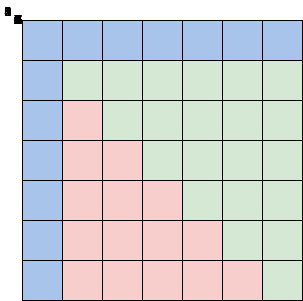Completed table[n][n]
1. Maintain a boolean table[n][n] filled from the bottom up.
2. If the substring is a palindrome, the value of table[i][j] is true. Otherwise the value is false.
3. To calculate table[i][j], we check the value of table[i+1][j-1]. If the value is true and str[i] is same as str[j], then the substring is a palindrome and we make table[i][j] true.
4. Otherwise, the value of table[i][j] is made false.
5. We then fill the table that was previously for the substring of length = 1 and length =2.

### Reimagine your coding interview prep

Stop grinding through endless practice questions, and start breaking down 100+ real-world problems. Practice as you learn with hands-on coding environments inside your browser. No need to switch to your IDE, download an SDK, or scrub through videos.

Decode the Coding Interview in C++: Real-World Examples

### 11. Reverse Words in a Sentence

Problem Statement

“Reverse the order of words in a given string.”

Hints:

• The problem asks for reversing word order, not letters within the word.
• Each word will be seperated by spacesExample Input and Output

Solution and Breakdown

void str_rev(char *str, int len) {

if (str == nullptr || len < 2) {
return;
}

char *start = str;
char *end = str + len - 1;

while (start < end) {
if (start != nullptr && end != nullptr) {
char temp = * start;
*start = *end;
*end = temp;
}
start++;
end--;
}
}

void reverse_words(char *sentence) {

// Here sentence is a null-terminated string ending with char '\0'.
if (sentence == nullptr) {
return;
}

// To reverse all words in the string, we will first reverse
// the string. Now all the words are in the desired location, but
// in reverse order: "Hello World" -> "dlroW olleH".

int len = strlen(sentence);
str_rev(sentence, len);

// Now, let's iterate the sentence and reverse each word in place.
// "dlroW olleH" -> "World Hello"

char *start = sentence;
char *end;
while (true) {
// find the  start index of a word while skipping spaces.
while (start && *start == ' ') {
++start;
}

if (start == nullptr || *start == '\0') {
break;
}

// find the end index of the word.
end = start + 1;
while (end && *end != '\0' && *end != ' ') {
++end;
}

// let's reverse the word in-place.
if (end != nullptr) {
str_rev(start, (end - start));
}

start = end;
}
}

int main() {
string str = "Red Car";
char *a = const_cast<char*>(str.c_str());

cout << a << endl;
reverse_words(a);
cout << a << endl;
}

Time Complexity: $O(n)$

The program works in two stages:

1. Reverse the entire string
2. Reverse each word in-place

Stage 2 works by setting a pointer for the beginning of the word, start, at the on element 0 or the first element after a space. We also set another pointer for the end of the word, end, on the element right before the next space.

Both pointers then iterate toward each other, swapping values on each element.

Once the pointers are on the same element, the word has be correctly reversed and our pointers move to the next word.

Our program is complete once all words have been reversed back to their proper order.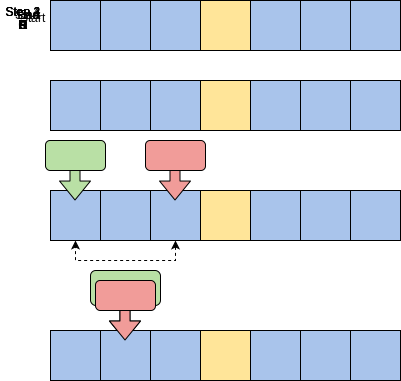Steps to reverse words in-place using pointers

### 12. Reverse First k Elements of Queue

Problem Statement

“Implement the function myQueue reverseK(myQueue queue, int k) which takes a queue and a number k as input and reverses the first k elements of the queue.”

Solution and Breakdown

main.cpp
stack.h
queue.h
#include "queue.h"
#include "stack.h"

myQueue reverseK(myQueue queue, int k) {
//1.Push first k elements in queue in a stack.
//2.Pop Stack elements and enqueue them at the end of queue
//3.Dequeue queue elements till "k" and append them at the end of queue
if (!queue.isEmpty()) {
myStack stack(k);
int count = 0;
while (count < k) {
stack.push(queue.dequeue());
count++;
}

while (!stack.isEmpty()) {
queue.enqueue(stack.pop());
}
int size = queue.getSize();

for (int i = 0; i < size - k; i++) {
queue.enqueue(queue.dequeue());
}

}

return queue;

}
int main(){
myQueue mQ(5);

mQ.enqueue(1);
mQ.enqueue(2);
mQ.enqueue(3);
mQ.enqueue(4);
mQ.enqueue(5);
mQ=reverseK(mQ,5);
mQ.showqueue(); //show queue prepended in the hidden code
return 0;
}

Time Complexity: $O(n)$

First we dequeue the first k elements from the front of the queue and push them in the stack we created on line 9 with stack.push(queue.dequeue()).

Once all the k values have been pushed to the stack, we start popping them and sequentially enqueuing them on line 14. We’ll queue them to the back using queue.enqueue(stack.pop()). At the end of this step, we will be left with an empty stack and the k reversed elements will be appended to the back of the queue.

Finally on line 19 we’ll move the resersed elements to the front of the queue usings queue.enqueue(queue.dequeue()). Each element is first dequeued from the back.

### 13. Zigzag Traversal

Problem Statement:

"Given a binary tree, populate an array to represent its zigzag level order traversal.

You should populate the values of all nodes of the first level from left to right, then right to left for the next level, alternating in the same way for all levels."

Hints:

• This question is an iteration of the “Binary Tree Level Order Traversal” question type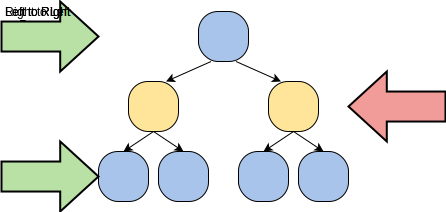Solution and Breakdown

using namespace std;

#include <iostream>
#include <queue>
#include <vector>

class TreeNode {
public:
int val = 0;
TreeNode *left;
TreeNode *right;

TreeNode(int x) {
val = x;
left = right = nullptr;
}
};

class ZigzagTraversal {
public:
static vector<vector<int>> traverse(TreeNode *root) {
vector<vector<int>> result;
if (root == nullptr) {
return result;
}

queue<TreeNode *> queue;
queue.push(root);
bool leftToRight = true;
while (!queue.empty()) {
int levelSize = queue.size();
vector<int> currentLevel(levelSize);
for (int i = 0; i < levelSize; i++) {
TreeNode *currentNode = queue.front();
queue.pop();

// add the node to the current level based on the traverse direction
if (leftToRight) {
currentLevel[i] = currentNode->val;
} else {
currentLevel[levelSize - 1 - i] = currentNode->val;
}

// insert the children of current node in the queue
if (currentNode->left != nullptr) {
queue.push(currentNode->left);
}
if (currentNode->right != nullptr) {
queue.push(currentNode->right);
}
}
result.push_back(currentLevel);
// reverse the traversal direction
leftToRight = !leftToRight;
}

return result;
}
};

int main(int argc, char *argv[]) {
TreeNode *root = new TreeNode(12);
root->left = new TreeNode(7);
root->right = new TreeNode(1);
root->left->left = new TreeNode(9);
root->right->left = new TreeNode(10);
root->right->right = new TreeNode(5);
root->right->left->left = new TreeNode(20);
root->right->left->right = new TreeNode(17);
vector<vector<int>> result = ZigzagTraversal::traverse(root);
cout << "Zigzag traversal: ";
for (auto vec : result) {
for (auto num : vec) {
cout << num << " ";
}
}
}


Time Complexity: $O(n)$

1. We start by pushing the root node to the queue.
2. We then iterate through the queue until the queue is empty.
3. In each iteration, we first count the elements in the queue (let’s call it levelSize). We will have this many nodes in the current level.
4. Next, remove levelSize nodes from the queue and push their value in an array to represent the current level.
5. After removing each node from the queue, insert both of its children into the queue.
6. If the queue is not empty, flip the direction of the traversal and repeat from step 3 for the next level.

### 14. Determine if an Undirected Graph is a Tree

Problem Statement:

“Create function bool isTree(Graph g) that takes a graph as input and returns if the passed graph is a tree.”

A graph is a tree if:

• There are no cycles.
• All nodes of the graph are reachable from all other nodes of the graph, directly or through traversal.

Hints:

• This can be solved using either Depth First Search or Breadth First Search

Solution and Breakdown

main.cpp
Graph.h
Node.h
#include "Graph.h"

using namespace std;

bool checkCycle(Graph g,int vertex, bool* visited, int parent)
{
// Mark the current vertex as visited
visited[vertex] = true;

// Recursive calls for all the vertices adjacent to this vertex

while (temp != NULL) {
// If an adjacent is not visited, then make recursive call on the adjacent
if (!visited[temp->data]) {
if(checkCycle(g,temp->data,visited,vertex))
return true;
}
else if (temp->data != parent)
return true;
//^ If an adjacent is visited and not parent of current
// vertex, then there is a cycle.
temp = temp->nextElement;
}

return false;
}

bool isTree(Graph g)
{

bool *visited = new bool[g.getVertices()];
for (int i = 0; i < g.getVertices(); i++)
visited[i] = false;

if (checkCycle(g,0, visited, -1))
return false;

for (int i = 0; i < g.getVertices(); i++) {
if (!visited[i])
return false;
}
delete[] visited;
visited = NULL;
return true;
}

int main() {
Graph g(5);

Graph g2(5);

cout << isTree(g) << endl;
cout << isTree(g2) << endl;

}

Time Complexity: $O(V+E)$

We have to check both conditions to determine if the graph is a tree.

To check the first condition, we start from the source and visit every adjacent vertex using recursive calls. If we come across any vertex that has already been visited and it is not the parent of the current vertex, then there is a cycle.

If we do not find such an adjacent for any vertex, we say that there is no cycle and the first condition is met.

For the second condition, we traverse all the vertices on the graph using recursive calls to check if they’re reachable from the source.

If we find any vertex that is not visited, we conclude that vertex is not reachable from the source. Therefore, the graph is not connected and does not met our second tree condition.

### 15. Word Formation From a Vector Using a Trie

Problem Statement:

“Implement the isFormationPossible() function, which finds whether a given word can be formed by combining two elements from a vector.”

main.cpp
Trie.h
#include "Trie.h"

bool isFormationPossible(vector<string> list,string word){
//Create Trie and insert vector elements in it
Trie * trie = new Trie();

for(int x = 0; x < list.size(); x++){
trie->insertNode(list[x]);
}

TrieNode * currentNode = trie->getRoot();

for (int i=0; i<word.size(); i++){
char index  = trie->getIndex(word[i]);
// if the prefix of word does not exist, word would not either
if (currentNode->children[index] == NULL){
return false;
}
// if the substring of the word exists as a word in trie, check whether rest of the word also exists, if it does return true
else if (currentNode->children[index]->isEndWord == true && trie->searchNode(word.substr(i+1))){
return true;
}
currentNode = currentNode->children[index];
}
return false;
}

int main() {
vector<string> keys = {"he", "hello", "loworld"};
if(isFormationPossible(keys, "helloworld"))
cout << "true\n";
else
cout << "false\n";

return 0;
}

Solution and Breakdown

Time Complexity: $O(m+n^2)$

First, we’ll make a trie using the words passed in the C++ vector.

Then, we check if there is a word in the trie which can become a part for the searched word. In the case of “helloworld”, we can find “he” in the trie.

Since there can be multiple elements that would serve as each part of a word, we have to check for applicable part.

If we find a part of the word that matches our searched word, we lookup the remaining word in the trie using the searchNode function.

If this substring exists within our searched word, we have found a solution.

## Recursion

### 16. Shuffle an Array of Integers

Problem Statement:

“Given an integer array of size n, create a program to recursively shuffle the array so no two elements remain next to each other. Do not use extra space in your solution.”

Hints:

• You can create the brute force solution first before refactoring to the recursive solution.
• Divide and Conquer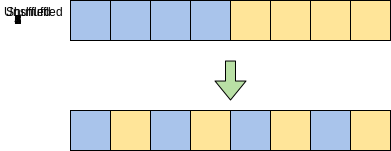Solution and Breakdown

#include <bits/stdc++.h>
using namespace std;

// Method to shuffle 2*n sized array's elements
void shuffleArrayRecursive(int left, int right, int arr[]) {
// Base case: return if 2 elements, no shuffle needed
if (right - left == 1)
return;

// get middle index of whole array
int middle = (left + right) / 2;

// get second half of first array
int mmiddle = (left + middle) / 2;

// get first half of second array
int temp = middle + 1;

// swapping elements for subarray
for (int i = mmiddle + 1; i <= middle; i++)
swap(arr[i], arr[temp++]);

// Pass divided first and second half to the
// recursive routine
shuffleArrayRecursive(left, middle, arr);
shuffleArrayRecursive(middle + 1, right, arr);
}

void shuffler(int a[], int n){
shuffleArrayRecursive(0, n-1, a);
}

int main() {
int a[] = { 1, 3, 5, 7, 2, 4, 6, 8 };
int n = sizeof(a) / sizeof(a);
shuffler(a, n);

for (int i = 0; i < n; i++)
cout << a[i] << " ";

return 0;
} 

Time Complexity: $O(nlogn)$

The program divides the given array into half (arr1[] and arr2[]) and then swaps the second element of arr1[] with the first element of arr2[]. Keep doing this recursively for all of arr1 and arr2.

We have a recursive utility function shuffleArrayRecursive() recursively called within the shuffler() function.

• Line 7-8: If there are only 2 elements, there is no need to do anything. This serves as the base case of our recursive call.
• Line 11-17: Divide the given array into two smaller subarrays of equal length, and find the middle of those subarrays.
• Line 20-21: Swap the elements from the right subarray (arr1[]) to the left subarray (arr2[]).
• Line 25-26: Make recursive calls to the function, with updated arguments, as calculated by finding the middle on Line 11-17.

### 17. Minimum Steps to Collect Coin Stacks

Problem Statement:

“Given an integer arrays representing the height of each stack of coins and the number of coin stacks, calculate the minimum number of straight lines that pass through all the coins (minimum number of steps to collect these coins).”

Hints:

• Divide and Conquer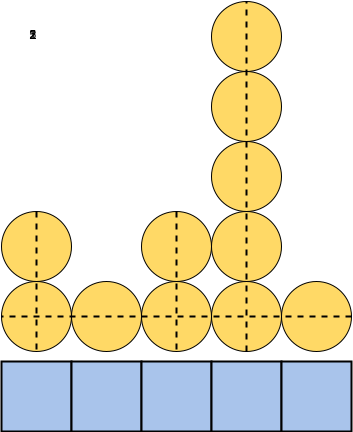Sample coin stacks with a minimum lines of 4

Solution and Breakdown

#include <iostream>
using namespace std;

// returns minimum of two numbers
int min2(int a, int b){
return a < b ? a : b;
}

/*
Utility method, called recursively to collect
coins from l to r using the height array
assuming that h height has already been collected
*/
int minimumStepsUtil(int l, int r, int h, int height[]) {
// base condition: all coins already collected
if (l >= r)
return 0;

// find minimum height index
int m = l;
for (int i = l; i < r; i++)
if (height[i] < height[m])
m = i;

/*
Calculate min steps by:
a) collecting all vertical line coins
(total r - l)
b) collecting all lower horizontal line coins
recursively on left and right segments
*/
return min2(r - l,
minimumStepsUtil(l, m, height[m], height) +
minimumStepsUtil(m + 1, r, height[m], height) +
height[m] - h);
}

/*
calls the recursive utility function
and returns the minimum number of steps
using height array
*/
int minimumSteps(int height[], int N) {
return minimumStepsUtil(0, N, 0, height);
}

// Testing minimumSteps() method
int main() {
int height[] = { 2, 1, 2, 5, 1 };
int N = sizeof(height) / sizeof(int);

cout << minimumSteps(height, N) << endl;
return 0;
}

Time Complexity: $O(n^2)$

First we start horizontally from the bottom, we can get rid of the lowest coin row and collect the maximum possible number of coins since the bottom rows are guaranteed to be filled. We’ll work on coin stacks from left, stackl, to right, stackr, in each recursion step.

Save the height of the smallest stack to m. Remove m horizontal lines, after which the stack will have two parts remaining: l to m and m + 1 to r. We’ll use these as subarrays.

We then make a recursive call on each subarray to repeat horizontal coin collection.

Since coins may also be collected using vertical lines, we then choose the minimum of the result of recursive calls and r – l.

Using (r – l) vertical lines, we can always collect all coins vertically.

### 18. Find the Number of Vowels in a String

Problem Statement:

“Create a function totalVowels that takes a string text parameter and returns the number of vowels within the string.”

Solution and Breakdown

#include <iostream>
#include <string>
using namespace std;

int totalVowels(string text, int len, int index)
{
int count=0;
if (len==0){return 0;}
char single=toupper(text[index]);
if(single=='A' || single=='E' || single=='I' || single=='O' || single=='U')
{
count++;
}
return count + totalVowels(text,len-1,index+1);
}

int main(){

cout<<"The string is: Hello World"<<endl;
cout<<"The total number of vowels in this string are: "<<totalVowels("Hello World",10,0)<<endl;

cout<<"The string is: STR"<<endl;
cout<<"The total number of vowels in this string are: "<<totalVowels("STR",3,0)<<endl;

cout<<"The string is: AEIOUaeiouSs"<<endl;
cout<<"The total number of vowels in this string are: "<<totalVowels("AEIOUaeiouSs",12,0)<<endl;

}

Time Complexity: $O(n)$

Provided that the length of the text string is not yet 0, we move to the recursive case. The first step in the function is to create a count variable, set to 0 which will be incremented each time a vowel is found.

To keep uniformity, we take the element of text at position index and convert it to uppercase. This reduces the different checks we will have in the subsequent if conditions. Then we equate the changed element to a variable, single to keep the code clean.

The if condition checks if single is equal to any of the vowels. If it is, then it increments count by 1.

After that the return statement simply calls on the recursive function again, totalVowels(), adding the result of the subsequent recursive call to the last value of count.

Note that this time, the len is reduced by 1 and the index is incremented by 1 to avoid an infinite loop.

## Dynamic Programming

### 19. Staircase Problem

Problem Statement:

“Given a stair with n steps, implement a method to count how many possible ways are there to reach the top of the staircase, given that, at every step you can either take 1 step, 2 steps, or 3 steps.”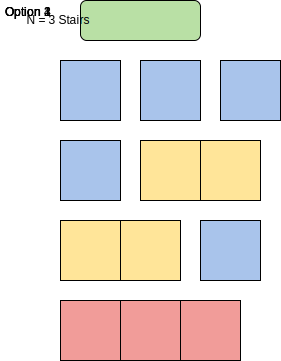Example solution for N = 3

Solution and Breakdown

using namespace std;

#include <iostream>
#include <vector>

class Staircase {

public:
int CountWays(int n) {
vector<int> dp(n + 1, 0);
return CountWaysRecursive(dp, n);
}

int CountWaysRecursive(vector<int> &dp, int n) {
if (n == 0) {
return 1; // base case, we don't need to take any step, so there is only one way
}

if (n == 1) {
return 1; // we can take one step to reach the end, and that is the only way
}

if (n == 2) {
return 2; // we can take one step twice or jump two steps to reach at the top
}

if (dp[n] == 0) {
// if we take 1 step, we are left with 'n-1' steps;
int take1Step = CountWaysRecursive(dp, n - 1);
// similarly, if we took 2 steps, we are left with 'n-2' steps;
int take2Step = CountWaysRecursive(dp, n - 2);
// if we took 3 steps, we are left with 'n-3' steps;
int take3Step = CountWaysRecursive(dp, n - 3);
dp[n] = take1Step + take2Step + take3Step;
}

return dp[n];
}
};

int main(int argc, char *argv[]) {
Staircase *sc = new Staircase();
cout << sc->CountWays(3) << endl;
cout << sc->CountWays(4) << endl;
cout << sc->CountWays(5) << endl;

delete sc;
}

Time Complexity: $O(n)$

This problem follows the Fibonacci number pattern.

The only difference is that in Fibonacci numbers every number is a sum of the two preceding numbers, whereas in this problem every count is a sum of three preceding counts. Here is the recursive formula for this problem:

CountWays(n) = CountWays(n-1) + CountWays(n-2) + CountWays(n-3), for n >=3


We also recognized that we’d have several overlapping subproblems and thus created a memoizaition table to save time.

### 20. Largest Sum Subarray

Problem Statement:

“Given an array, find the contiguous subarray with the largest sum.”

Hints:

• Your program only needs to return the sum of the values, not the indexes of the subarray.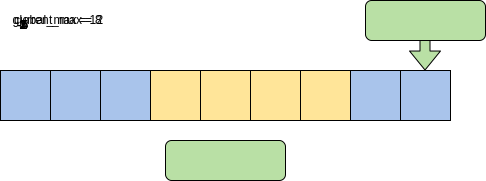Example array at completion

Solution and Breakdown

using namespace std;

#include <iostream>

int find_max_sum_sub_array(int A[], int n) {
if (n < 1) {
return 0;
}

int curr_max = A;
int global_max = A;
for (int i = 1; i < n; ++i) {
if (curr_max < 0) {
curr_max = A[i];
} else {
curr_max += A[i];
}

if (global_max < curr_max) {
global_max = curr_max;
}
}

return global_max;
}

int main() {

int v[] = {-4, 2, -5, 1, 2, 3, 6, -5, 1};
cout << "Sum of largest subarray: " << find_max_sum_sub_array(v, sizeof(v) / sizeof(v)) << endl;
return 0;
}

Time Complexity: $O(n)$

Kadane’s algorithm scans the entire array and at each position finds the maximum sum of the subarray ending there.

This is achieved by keeping a current_max summing up to the current index and a global_max that contains the largest sum found by that point. Whenever current_max is greater than global_max, we set global_max to that new highest value.

Since the values must be contiguous, we can discontinue any possible combination if elements if the sum of the current subarray goes down i.e. we encounter a negative number.

## 20 more C++ interview questions

1. Rod Cutting Problem
2. Rotate an Array of N Elements
3. Stock Buy Sell to Maximize Profits
4. Cyclic Sort
5. Find K-Sum Subsets
6. Search in a Sorted Infinite Array
7. Reverse Alternate K Nodes in a Singly Linked List
8. Kth Smallest Number in M Sorted Lists
9. Find the Missing Number in a Linked List
10. Minimum Deletions in a String to create a Palindrome
1. Balanced Parentheses Problem
2. Longest Substring with K Distinct Characters
3. Convert Binary Tree to Doubly Linked List
4. Sliding Window Median Problem
5. Topological Sort a Graph
6. Alien Dictionary Problem
7. Prime Number Checker
8. Find the Closest Pair of Points in Euclidean Space
9. Knapsack Problem
10. Equal Subset Sum Partition Problem

## Wrapping up and next steps

Congratulations on finishing those 40 questions!

The best way to prepare for coding interviews is the practice you’re doing right now. Soon, you’ll know all the question types you could encounter at your next interview.

To help you prepare for interviews, Educative has created the unique course Decode the Coding Interview in C++: Real-World Examples. Available in multiple languages, this course takes you through 100+ real-world questions like these. After each project, you’ll better understand what techniques you just applied.

This is coding interview prep reimagined, with your needs in mind

Happy learning!